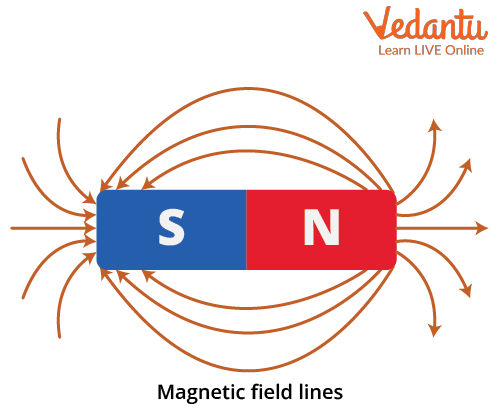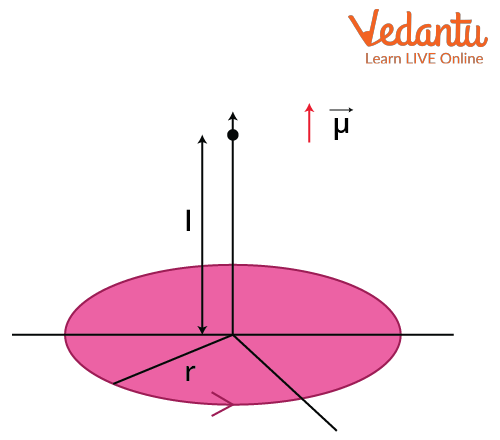Courses
Courses for Kids
Free study material
Free LIVE classes
More

# Current Loop as Magnetic Dipole and Its Derivation for JEELIVE
Join Vedantu’s FREE Mastercalss

## Introduction to Magnetic Dipoles

A magnetic dipole is formed by taking a magnetic north pole and south pole closer and closer together, keeping the product of the magnetic pole charge and distance constant. The idea that a current loop can act as a magnetic dipole was given by Ampere. He explained the phenomenon with the help of the circular movement of current through the wire. It is almost similar to the magnetic dipole with the magnetic field line straight at the centre of the loop and a curvy closed loop all over, and the direction of the lines is determined by the direction of current flow in the wired loop. To understand the dipole due to current flow in a loop, we will discuss it in detail below.

## Magnetic Dipole and Magnetic Dipole Moment

As we know, a magnetic dipole is a set-up where two opposite magnetic charges are kept at a very short distance. For example, a bar magnet.

The magnetic dipole moment is defined as the maximum amount of torque generated by magnetic force on a dipole that arises per unit value of the surrounding magnetic field in a vacuum. It is a vector quantity denoted by 𝜇 and its direction is from the north pole to the south pole. It has the unit Ampere - meter2 in SI and ergs per gauss and 1 Ampere - meter2 is equal to 1000 ergs per gauss.

We have the torque of the dipole by the formula,

$\tau=M \times B$

Where,

• M = magnetic moment

• B = magnetic fieldImage: Magnetic dipole( Bar magnet) and field lines

## Current Loop as a Magnetic Dipole and Its Dipole Moment

If current passes through a circular loop, it acts as a magnetic dipole as a magnetic field is generated due to the flow of charges. It acts through the geometry of the loop that tries to align the magnetic object around it and creates a magnetic field or magnetic field lines accordingly.

Let us define the magnetic dipole moment in the current loop. The magnetic dipole moment of a current loop is the product of the current passing through the loop and the area inside the loop. So, the magnetic dipole moment formula is,

$\mu=n I A$

where,

• n = number of turns in the loop

• I = current in the loop

• A = area inside the loop

The direction of the dipole moment can be determined by the right hand thumb rule.Image: Right hand thumb rule applied on current loop

## Circular Current Loop as a Magnetic Dipole

As Ampere has observed, the circular current loop acts as a magnetic field due to the current moving in the circular direction or in a loop and its direction is determined according to the “right hand thumb” rule. Now we will try to derive some formulas. So, the formula for a circular current loop is,

$\mu=n i \pi r^{2}$

Where,

• n= number of turns

• i= current flowing

• r= radius of the loop

## Current Loop as a Magnetic Dipole Derivation

Here, we will use a circular loop but it can be generalised using the formula of dipole moment. Consider a circular loop with radius “r” lying on the table, which is carrying a current “i” in an anti-clockwise direction. Now, we have a point P that is “l” distance apart from the centre of the loop. So, the magnetic field at p isImage: Magnetic field of current loop

$B=\dfrac{\mu_{0}{i r^{2}}}{2\left(r^{2}+l^{2}\right)^{\dfrac{3}{2}}}$

Now, consider P is much much greater than the radius of the loop,

We can estimate the field as,

$B=\dfrac{\mu_{0}{i r^{2}}}{2 l^{3}\left(\left(\dfrac{r}{l}\right)^{2}+1\right)^{\dfrac{3}{2}}} \approx \dfrac{\mu_{0} i r^{2}}{2 l^{3}}=\dfrac{\mu_{0}}{4 \pi} \dfrac{2 i\left(\pi r^{2}\right)}{l^{3}}$

as, $r << l$

Now we have, area of the loop,

$A=\pi r^{2}$

So, the magnetic field is,

$B=\dfrac{\mu_{0}}{4 \pi} \dfrac{2 i A}{l^{3}}=\dfrac{\mu_{0}}{4 \pi} \dfrac{2 \mu}{l^{3}}$

As, $\mu=iA$

This quantity $\mu$ is a vector quantity and we can implement that in above formula,

$\vec{B}=\dfrac{\mu_{0}}{4 \pi} \dfrac{2 \vec{\mu}}{l^{3}}$.........(a)

It is just like the formula of electric dipole field,

$\vec{E}=\dfrac{1}{4 \pi \varepsilon_{0}} \dfrac{2 \vec{p}}{r^{3}}$.......(b)

Therefore, upon comparing the equation (a) and (b), we can say that $\vec{\mu}$ is known as the magnetic dipole moment.

## Magnetic Moment Inside an Atom

In an atom, electrons continuously revolve around the nucleus and thus current is generated around the nucleus. It is similar to a circular loop. So, we can calculate the magnetic moment of the inside atom due to the rotation of electrons.

The current due to rotation of electron is,

$i=\dfrac{e}{T}=\dfrac{e}{\dfrac{2 \pi R}{v}}=\dfrac{e v}{2 \pi R}$

e= charge of electron

v= velocity of electron

So, magnetic moment,

$\mu=I A=\dfrac{e v}{2 \pi R} \pi R^{2}=\dfrac{e v R}{2}$

We have the angular momentum as,

\begin{align} &L=m_{e} v R \\ &\Rightarrow v R=\dfrac{L}{m_{e}} \end{align}

So,

\begin{align} &\mu=\dfrac{e L}{2 m_{e}} \\ &\Rightarrow \dfrac{\mu}{l}=\dfrac{e}{2 m_{e}} \end{align}

The above equation is called Gyromagnetic ratio. The magnetic moment of electrons is measured by Bohr-Magneton and it is equivalent to 9.27 $\times$ 10-24 ampere -m2.

## Conclusion

So, here we get to see that a current-carrying loop can act like a bar magnet. We know that a conducting wire with the current flow can generate the magnetic field, i.e., can generate magnetic field lines around it, and as it is now looped, it goes in a particular way, making closed loops of magnetic field lines that is similar to the bar magnet system. We can also observe this in fundamental particles like electrons, and these principles are used in making Hadron Colliders and imaging instruments (MRI).

Last updated date: 22nd Sep 2023
Total views: 133.8k
Views today: 3.33k

## FAQs on Current Loop as Magnetic Dipole and Its Derivation for JEE

1. How does a magnetic moment cause paramagnetism?

We know that matter is made of atoms and that electrons move around the nucleus, creating a magnetic moment. So, we can consider each atom as a little bar magnet. So, any matter consists of little bar magnets. Now, while the formation of the entire matter, the bar magnets align randomly, thus creating the net magnetic moment zero. For some matters, these are aligned in particular patterns, creating a non-zero magnetic moment. This phenomenon is called paramagnetism. Likewise, it gets aligned in a particular direction while being charged by an external magnetic field.

2. How can we calculate the magnetic field of squared or any shape loop?

Firstly the formula is given, $\mu=n I A$, so we can use formula directly to get the magnetic moment and then the field associated to it just by using formula

$\vec{B}=\dfrac{\mu_{0}}{4 \pi} \dfrac{2 \vec{\mu}}{l^{3}}$.

Now, if you want to calculate it manually without using the formula here are few steps you can follow:

• First, align the loop on the X-Y plane with the current in an anti-clockwise direction.

• Then choose a point on the Z-axis at a distance much greater than the dimension of the loop.

• Then use geometric intuitions to calculate the Field vector. From there you can get the moment too. (There will be a lot of component vectors cancelling each other. Look for those ones.)